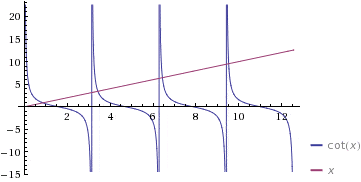# Tan x = 1/x

How do I find the first 10 positive solutions numerically?
$$\tan x = \frac{1}{x}$$

I tried using NSolve in Mathematica but that didn't work.

I also tried
syms x
solve(tan(x)==1/x)
in Matlab and that just gave -263.

How can I do this in one of these programs?

Last edited:
Ray Vickson
Homework Helper
Dearly Missed
How do I find the first 10 positive solutions numerically?
$$\tan x = \frac{1}{x}$$

How do you find the first positive solution? You tell US; do not ask us to tell you: read the forum rules.

RGV

Last edited:
SammyS
Staff Emeritus
Homework Helper
Gold Member
How do I find the first 10 positive solutions numerically?
$$\tan x = \frac{1}{x}$$
Where are you stuck?

What have you tried?

After 490 posts, you should know the drill here at PF .

But here's one hint: One thing I would try is to take the reciprocal of both sides of that equation, giving you:
$\displaystyle \cot(x)=x$​

Graph each side.

How do you find the first positive solution? You tell US; do not ask us to tell you: read the forum rules.

RGV

I am not good at numerical analysis. I haven't taken a course in it yet as well as in Matlab. I will be taking Matlab this spring and Numerical Analysis next fall. So when I know how to do it and use Matlab efficiently, I won't even ask you.

Where are you stuck?

What have you tried?

After 490 posts, you should know the drill here at PF .

But here's one hint: One thing I would try is to take the reciprocal of both sides of that equation, giving you:
$\displaystyle \cot(x)=x$​

Graph each side.

Numerically means approximately since this is a transcendental equation. Only some computer software would give you the results, I think.

http://www.wolframalpha.com/input/?i=Plot+y(x)+=+tan+x+-+1/x

I have a graph of it. I don't know if the forum can handle tikz but here it is
$$\begin{tikzpicture}[>=stealth',x = .5cm,y = .5cm,scale = .60] \def\npi{3.1416} \def\periods{4} \draw[->] (-\npi/2,0) -- ({(\periods + .5)*\npi},0) node[above] {\lambda_n}; \draw[->] (0,-10) -- (0,10) node {f(\lambda_n)}; \clip (-\npi/2,-9.8) rectangle ({(\periods + .5)*\npi},9.8); \draw[thick, domain = 0.05:{(\periods + .4)*\npi},samples = 300,smooth,color = red] plot (\x,1/\x); \foreach \n in {0,...,4} \draw[thick,shift = {(\npi*\n,0)},domain = -\npi/2+.1:\npi/2-.1,samples = 100,smooth] plot (\x,{tan(\x r)}); %draw the ticks \foreach \x in {1,...,10} \draw (\x*\npi/2,2pt) -- (\x*\npi/2,-2pt); %draw labels n\pi/2 for odd n >= 3 \foreach \x in {3,5,...,7} \node[below] at (\x*\npi/2,0) {\frac{\x\pi}{2}}; %draw labels n\pi for n >= 2 \foreach \x in {2,...,4} \node[below] at (\x*\npi,0) {\x\pi}; \node[below] at (\npi/2,0) {\frac{\pi}{2}}; \node[below] at (\npi,0) {\pi}; \end{tikzpicture}$$​

Ray Vickson
Homework Helper
Dearly Missed
I am not good at numerical analysis. I haven't taken a course in it yet as well as in Matlab. I will be taking Matlab this spring and Numerical Analysis next fall. So when I know how to do it and use Matlab efficiently, I won't even ask you.

You don't need to know how to use Matlab, etc. Just apply Newton's method, which you can do using a hand-held scientific calculator. If you have not ever seen Newton's method before, I would be very surprised.

RGV

SammyS
Staff Emeritus
Homework Helper
Gold Member
Here are the graphs of y=x and y=cot(x), superimposed. (from WolframAlpha)#### Attachments

Here are the graphs of y=x and y=cot(x), superimposed. (from WolframAlpha)I tried using NSolve in Mathematica but that didn't work.

I also tried
syms x
solve(tan(x)==1/x)
in Matlab and that just gave -263.

How can I do this in one of these programs?
This is what I wrote in post 3.
I can make graphs of this with the Tikz package and in Mathematica (that isn't the challenge here). How can I use Mathematica or Matlab to generate the first 10 positive solution?

Ray Vickson
Homework Helper
Dearly Missed
I tried using NSolve in Mathematica but that didn't work.

I also tried
syms x
solve(tan(x)==1/x)
in Matlab and that just gave -263.

How can I do this in one of these programs?
This is what I wrote in post 3.
I can make graphs of this with the Tikz package and in Mathematica (that isn't the challenge here). How can I use Mathematica or Matlab to generate the first 10 positive solution?

I don't have access to Mathematica (except through Wolfram Alpha) or to Matlab, but in Maple it is easy:
S:=fsolve(tan(x)=1/x,x=0..Pi/2),seq(fsolve(tan(x)=1/x,x=Pi/2+(i-1)*Pi..Pi/2+i*Pi),i=1..9);
S := 0.8603335890, 3.425618459, 6.437298179, 9.529334405,

12.64528722, 15.77128487, 18.90240996, 22.03649673,

25.17244633, 28.30964285
I'm sure it must be possible, even easy, to do the same thing in Mathematica.

RGV

Last edited:
I don't have access to Mathematica (except through Wolfram Alpha) or to Matlab, but in Maple it is easy:
S:=fsolve(tan(x)=1/x,x=0..Pi/2),seq(fsolve(tan(x)=1/x,x=Pi/2+(i-1)*Pi..Pi/2+i*Pi),i=1..9);
S := 0.8603335890, 3.425618459, 6.437298179, 9.529334405,

12.64528722, 15.77128487, 18.90240996, 22.03649673,

25.17244633, 28.30964285
I'm sure it must be possible, even easy, to do the same thing in Mathematica.

RGV
Thanks. Hopefully in the spring when I take programming in Matlab I will be better at this.

vela
Staff Emeritus
Homework Helper
I tried using NSolve in Mathematica but that didn't work.

I tried using NSolve in Mathematica but that didn't work.
...
How can I do this in one of these programs?

In:= Plot[Tan[x]-1/x,{x,.01,30}]

Out= <snip graphic showing approximate positions of solutions>

In:= FindRoot[Tan[x]-1/x,{x,.9}]

Out= {x->0.860334}

In:= FindRoot[Tan[x]-1/x,{x,3.5}]

Out= {x->3.42562}

etc,etc,etc